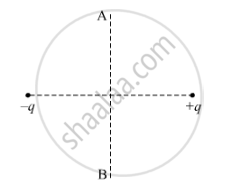# Find the Work Done in the Process. - Physics

A charge 'q' is moved from a point A above a dipole of dipole moment 'p' to a point B below the dipole in equatorial plane without acceleration. Find the work done in the process.#### Solution

The net force on the charge q is directed parallel to the dipole and hence perpendicular to its motion along AB.

∴ Work done = 0

Concept: Magnetic Dipole Moment of a Revolving Electron
Is there an error in this question or solution?